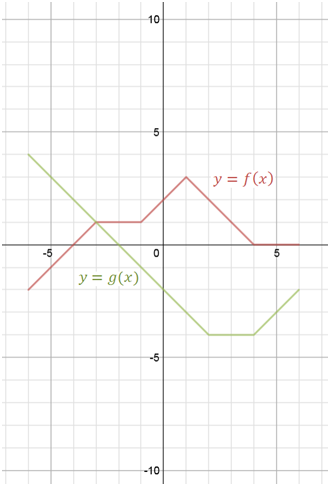# Multiplying functions

### Multiplying functions

#### Lessons

multiplying functions: $(fg)(x) = f(x)\cdot g(x)$
• 1.
Determine the Product of Two Functions Algebraically
If $f(x)= 2-\sqrt{x}$
and
$g(x)=7\sqrt{x}-3$
Determine:
a)
$(fg)(x)$

b)
$(fg)(25)$
evaluate in two different ways

• 2.
Determine the Product of Two Functions Graphically
Given the graph of functions $f(x)$
and $g(x)$,
sketch the product of these two functions$(fg)(x)$